# KR101090510B1 - Control and Error correction method of angular position estimation for Permanent Magnet Synchronous Motors - Google Patents

Control and Error correction method of angular position estimation for Permanent Magnet Synchronous Motors Download PDF

## Info

Publication number
KR101090510B1
KR101090510B1 KR1020090072457A KR20090072457A KR101090510B1 KR 101090510 B1 KR101090510 B1 KR 101090510B1 KR 1020090072457 A KR1020090072457 A KR 1020090072457A KR 20090072457 A KR20090072457 A KR 20090072457A KR 101090510 B1 KR101090510 B1 KR 101090510B1
Authority
KR
South Korea
Prior art keywords
rotor
error correction
estimated
calculated
correction value
Prior art date
Application number
KR1020090072457A
Other languages
Korean (ko)
Other versions
KR20110014874A (en
Inventor
김삼영
이우택
최진철
Original Assignee
창원대학교 산학협력단
Priority date (The priority date is an assumption and is not a legal conclusion. Google has not performed a legal analysis and makes no representation as to the accuracy of the date listed.)
Filing date
Publication date
Application filed by 창원대학교 산학협력단 filed Critical 창원대학교 산학협력단
Priority to KR1020090072457A priority Critical patent/KR101090510B1/en
Publication of KR20110014874A publication Critical patent/KR20110014874A/en
Application granted granted Critical
Publication of KR101090510B1 publication Critical patent/KR101090510B1/en

• 230000001360 synchronised Effects 0.000 title claims abstract description 40
• 230000001276 controlling effects Effects 0.000 claims description 11
• 238000004364 calculation methods Methods 0.000 claims description 8
• 230000000875 corresponding Effects 0.000 claims 2
• 238000010586 diagrams Methods 0.000 description 10
• 239000002529 flux Substances 0.000 description 10
• 230000004907 flux Effects 0.000 description 10
• 241001124134 Chrysomelidae Species 0.000 description 4
• 238000004804 winding Methods 0.000 description 3
• 238000006243 chemical reactions Methods 0.000 description 2
• 230000000694 effects Effects 0.000 description 2
• 230000037010 Beta Effects 0.000 description 1
• 230000005355 Hall effect Effects 0.000 description 1
• 280000867207 Lambda companies 0.000 description 1
• 230000001939 inductive effects Effects 0.000 description 1
• 238000000034 methods Methods 0.000 description 1
• 230000000051 modifying Effects 0.000 description 1

## Images

•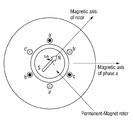•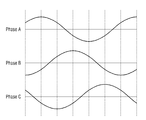•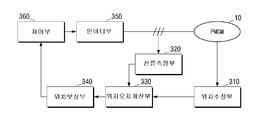•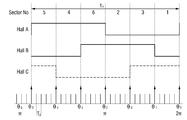•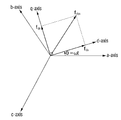•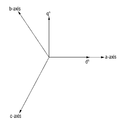•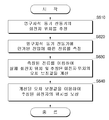••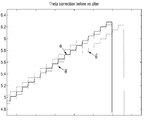•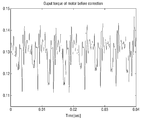•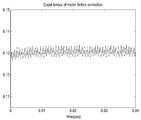## Classifications

• HELECTRICITY
• H02GENERATION; CONVERSION OR DISTRIBUTION OF ELECTRIC POWER
• H02PCONTROL OR REGULATION OF ELECTRIC MOTORS, ELECTRIC GENERATORS OR DYNAMO-ELECTRIC CONVERTERS; CONTROLLING TRANSFORMERS, REACTORS OR CHOKE COILS
• H02P6/00Arrangements for controlling synchronous motors or other dynamo-electric motors using electronic commutation dependent on the rotor position; Electronic commutators therefor
• H02P6/14Electronic commutators
• H02P6/16Circuit arrangements for detecting position
• HELECTRICITY
• H02GENERATION; CONVERSION OR DISTRIBUTION OF ELECTRIC POWER
• H02KDYNAMO-ELECTRIC MACHINES
• H02K21/00Synchronous motors having permanent magnets; Synchronous generators having permanent magnets
• H02K21/02Details
• H02K21/10Rotating armatures
• HELECTRICITY
• H02GENERATION; CONVERSION OR DISTRIBUTION OF ELECTRIC POWER
• H02PCONTROL OR REGULATION OF ELECTRIC MOTORS, ELECTRIC GENERATORS OR DYNAMO-ELECTRIC CONVERTERS; CONTROLLING TRANSFORMERS, REACTORS OR CHOKE COILS
• H02P2203/00Indexing scheme relating to controlling arrangements characterised by the means for detecting the position of the rotor
• H02P2203/03Determination of the rotor position, e.g. initial rotor position, during standstill or low speed operation

## Abstract

A permanent magnet synchronous motor control system is disclosed. The permanent magnet synchronous motor control system includes a position estimator for estimating the rotor position of the motor, a current measuring unit for measuring a current according to a voltage applied to the motor, and an actual rotor position and estimated rotation using the measured current. And a position error calculator for calculating an error correction value of the former position, and a position compensator for compensating the estimated position of the rotor using the calculated error correction value. Accordingly, the performance of the motor can be improved.
Permanent Magnet Synchronous Motor, Rotor Position, Error Compensation

## Description

Control and Error correction method of angular position estimation for Permanent Magnet Synchronous Motors

The present invention relates to a permanent magnet synchronous motor control system and a control method thereof, and more particularly to a permanent magnet synchronous motor control system and a control method for controlling the motor by compensating the rotor position.

Permanent magnet synchronous motor is one of the motors currently used in various fields because of its high efficiency and high output density even in the high speed range. It uses a permanent magnet with a sine wave shape as an internal rotor and an external stator. Is a motor wound three-phase winding with the number of windings distributed in sine wave form.

In order to drive such a permanent magnet synchronous motor, the position information of the rotor is necessarily required, and an encoder having a high resolution as a position sensor is mainly used to detect this information.

However, encoders are not suitable for high-speed driving due to their structural constraints and weak mechanical stiffness, and they are also relatively expensive compared to other sensors.

As an alternative to such an encoder, sensorless control without using a position sensor has been proposed, but this also has a problem that accurate position estimation is difficult in a low speed region.

In order to solve this problem, a method of using a hall sensor as a position sensor has been proposed.

However, there is a problem in that an error occurs in the estimation of the position of the rotor due to the asymmetrical arrangement of the Hall sensors and the jitter of the Hall sensor signals.

SUMMARY OF THE INVENTION The present invention has been made to solve the above problems, and an object of the present invention is to provide a permanent magnet synchronous motor control system and a control method for controlling the motor by using the compensated rotor position.

According to an embodiment of the present invention, the permanent magnet synchronous motor control system, the position estimation to estimate the rotor position of the motor, the current measuring unit for measuring the current according to the voltage applied to the motor, by using the measured current And a position error calculator for calculating an error correction value of the actual rotor position and the estimated rotor position, and a position compensator for compensating the estimated rotor position using the calculated error correction value.

The apparatus may further include an inverter unit for outputting a driving signal for driving the electric motor, and a controller for outputting a control signal for controlling the inverter unit, wherein the controller is configured to operate the inverter using the compensated rotor position. You can control wealth.

The position estimator estimates the angular velocity of the rotor, and the position error calculator calculates the position of the actual rotor using the command voltage from the controller, the measured current, and the estimated angular velocity. The error correction value may be calculated using the actual rotor position and the estimated rotor position.

Here, the error correction value of the actual rotor position and the estimated rotor position may be calculated as a product of a vector of a unit back electromotive force function for each rotor.

The position estimator may include a hall sensor for estimating the position of the rotor.

On the other hand, the permanent magnet synchronous motor control method according to an embodiment of the present invention, estimating the rotor position of the motor, measuring the current according to the voltage applied to the motor, the actual using the measured current Calculating an error correction value of the rotor position and the estimated rotor position, and compensating the estimated rotor position using the calculated error correction value.

The method may further include outputting a drive signal for driving the electric motor by the inverter, and outputting a control signal for controlling the inverter by the controller, wherein the controller uses the position of the compensated rotor. To control the inverter.

The step of estimating the rotor position may include estimating the angular velocity of the rotor, and calculating the error correction value by using the command voltage, the measured current, and the estimated angular velocity in the controller. The position of the former may be calculated, and the error correction value may be calculated using the position of the actual rotor and the estimated position of the rotor.

In this case, an error correction value of the actual rotor position and the estimated rotor position may be calculated as a product of a vector of unit back electromotive force functions for each rotor.

The motor may include a hall sensor for estimating the position of the rotor.

Accordingly, it is possible to improve the permanent magnet synchronous motor control performance and increase the efficiency of the system.

Hereinafter, with reference to the accompanying drawings will be described in detail with respect to the present invention.

1 is a schematic diagram showing the structure of a permanent magnet electric motor to help understanding of the present invention.

The permanent magnet synchronous motor shown in FIG. 1 uses a permanent magnet having a magnetic flux intensity in the form of a sinusoidal wave as an internal rotor, and an external stator is a motor wound three-phase winding in which the number of windings is distributed in the form of a sinusoidal wave.

Unlike DC motors, permanent magnet synchronous motors do not have commutators or brushes, so they can be used semi-permanently without maintenance as long as the motor itself is not worn out or broken. And high efficiency. In addition, high efficiency and power factor are advantages compared to induction motors.

Briefly explaining the driving method of the permanent magnet synchronous motor as follows. The stator three-phase sinusoidal current forms a rotating magnetic field rotating at the synchronous angular velocity as shown in Equation (1).

In the rotor, torque is generated by the magnetic force between the magnetic flux caused by the permanent magnet and the rotor magnetic field. As a result, the permanent magnet synchronous motor is driven.

2 is a view for explaining the form of back EMF for better understanding of the present invention.

As shown in FIG. 2, the counter electromotive force of the permanent magnet synchronous motor is a reverse voltage induced in the stator winding by changing the magnetic flux of the permanent magnet due to the rotation of the rotor. This is obtained by applying Faraday's law to each phase as shown in Equation 2.

Here, e as , e bs , and e cs are back EMFs generated on A, B, and C, respectively, and λ as , λ bs , and λ cs are magnetic fluxes in units in which the A, B, and C phase windings are wound. The minus sign simply means that the current generated by the counter electromotive force produces a magnetic flux opposite to the magnetic flux that caused the counter electromotive force. In the permanent magnet synchronous motor, the counter electromotive force has a sinusoidal shape as shown in FIG. 2.

Meanwhile, in order to control the permanent magnet synchronous motor, the counter electromotive force information of the permanent magnet synchronous motor is used. The reason is that the rectifier is

This is because the motor can maintain a constant torque only when the relative position of the rotor and the stator is known and rectified at the relative position. Therefore, for torque control of the permanent magnet synchronous motor, it is necessary to feed back the rotor position of the permanent magnet synchronous motor.

3 is a block diagram showing the configuration of a permanent magnet synchronous motor control system according to an embodiment of the present invention.

According to FIG. 3, the permanent magnet synchronous motor control system 300 includes a permanent magnet synchronous motor (PMSM) 10, a position estimation 310, a current measurement unit 320, and a position error calculation unit. 330, a position compensator 340, an inverter 350, and a controller 360.

The PMSM 10 includes a magnetic pole position sensor, and may be a Hall-effect sensor.

For example, three Hall sensors can be mounted with a 120 degree phase difference around the motor rotor.

In addition, since the description of the PMSM 10 has been described above with reference to FIG. 1, a detailed description thereof will be omitted.

The position estimator 310 estimates the position of the rotor through the state of a Hall sensor (not shown) signal generated when the PMSM 10 rotates. The position estimation of the rotor will be described in detail with reference to FIG. 4.

The current measuring unit 320 measures the current in the PMSM 10. Here, the measured current may be a current on the two-phase fixed coordinate system ds axis (hereinafter referred to as α) and qs axis (hereinafter referred to as β).

Position error calculation unit 330 is the current measured by the current measuring unit 320

And the voltage command value from the controller 360 to be described later. , The angular velocity estimated by the position estimation unit 310 Error between the estimated rotor position and the actual rotor position Calculate

The compensation unit 340 estimates the position of the rotor estimated by the position estimating unit 310.

Error value calculated in the position error calculation unit 330 Corrected value by correcting Is calculated and provided to the control unit 360.

The inverter unit 350 is a power conversion device that converts DC power into AC power. The inverter unit 350 may be implemented as a voltage inverter, but in some cases, the inverter unit 350 may be implemented as a current inverter.

The control unit 360 functions to output a control signal for controlling the inverter unit 350.

The controller 360 may be implemented as a controller based on Space Vector Pulse Width Modulation (SVWWM), and outputs a PWM control signal for controlling the inverter unit 350. Here, SVPWM is based on converting three-phase physical quantities into two-phase fixed coordinate system physical quantities.

In general, the stator three-phase voltage equation of a permanent magnet synchronous motor is represented by a complex differential equation having a time-varying coefficient. Obtaining this differential equation is very complicated. Therefore, the reference coordinate system theory is introduced to make time-varying elements into constant elements. Variable conversion is used to more easily solve complex time-varying differential equations for three-phase voltage equations.

In addition, the controller 360 may receive a feedback of the rotor position compensated by the compensator 340 and output a current control signal for driving the inverter 350.

4 is a view for explaining the principle of rotor position estimation according to an embodiment of the present invention.

According to Fig. 4, one period of the signal is divided into six sectors (sector Nos. 1-6), and an absolute position θs of the rotor is given to each sector.

As shown in Equation 3, the time for one period is calculated using the electrical angle angular velocity w h using t h .

Subsequently, Δθ, which is a small change amount of the rotor position, is calculated using the angular velocity and the micro time Ts calculated in Equation 3 as shown in Equation 4.

Subsequently, as shown in Equation 5, the position of the rotating child can be estimated while increasing the amount of small change Δθ calculated in Equation 4 from the absolute position?

5A and 5B are diagrams illustrating a relationship between a three-phase coordinate system and a two-phase fixed coordinate system for better understanding of the present invention.

It is a figure which shows the relationship between the three-phase coordinate system and the 2-phase coordinate system (alpha)-(beta) axis (d-q axis | shaft) which rotates by arbitrary speed w.

Here, the meaning of what is represented by f jk i is defined as follows. f expresses voltage, current or magnetic flux. The superscript i represents the type of coordinate system, in the case of "i = e" the synchronous coordinate system (w = w e ), in the case of "i = r" the rotor coordinate system (w = w r ), and "i = s ", the stop coordinate system (w = w s ) is displayed. Subscript j denotes an α-axis variable when "j = d", β-axis variable when "j = q", and n-axis variable when "j = n". The subscript k denotes the stator quantum in the case of "k = s" and the rotor quantum in the case of "k = r".

The relation between the function f dqs of the two-phase coordinate system rotating at an arbitrary speed w and f representing a three-phase variable as a vector is expressed by Equation 6.

Where a = e j2 π / 3 , a 2 = e j4π / 3 = e - j2π / 3

The two-phase fixed coordinate system is a two-phase coordinate system in which the two-phase coordinate system d-axis coincides with the three-phase coordinate system a phase with θ = 0, as shown in FIG. 6B.

The relationship between the two-phase fixed coordinate system and the three-phase vector is obtained by substituting θ = 0 in Equation 6, as shown in Equation 7.

Hereinafter, the calculation process of the position error calculation unit 330 will be described.

Position error calculation unit 330 is the current measured by the current measuring unit 320

And the voltage command value from the controller 360 to be described later. , The angular velocity estimated by the position estimation unit 310 Error between the estimated rotor position and the actual rotor position Calculate Here, the voltage command value from the control unit 360 Is the voltage at the motor Same as the current measured in the current measuring unit 320 Current at the motor Assume the same as

First, a voltage relation equation is obtained on a two-phase fixed coordinate system, as shown in Equation 8.

Here, Rs is a resistance component, and λ α and λ β are the amount of stator chain bridge magnetic flux on the α axis and the β axis, respectively.

On the other hand, the amount of stator linkage flux λ α and λ β on the α axis and β axis are as shown in Equation (9).

Where Ls is a magnetic inductance component and lambda m is a constant.

Using Equations 8 and 9, the voltages u α and u β on the α-axis and the β-axis are rearranged as shown in Equation 10.

Where ρ is the derivative operator.

The voltages u α , u β that are actually applied to the motor and u * α , u * β , which are command values from the control unit 360, are expressed by Equation 11 below.

here,

ego, to be.

Using Equation (11), the linkage flux increase and decrease amounts Δλ α and Δλ β can be summarized as in Equation 12.

Estimated rotor position

And actual rotor position Error In this case, Equation 13 is established between them.

In addition, Equation 12 is a unit vector

Wow Is summarized as in Equation (14).

here,

Can be calculated from Δθ, λ m , Δλ α , Δλ β , Is the estimated rotor position Can be calculated from

Also, unit vector

Wow When the product cross product (Outer Product or Cross Product),

That is, the estimated rotor position

And actual rotor position Error Is a very small value, the last term of Eq. Wow Error from the cross product of Will be available.

The position value of the rotor corrected accordingly

Equation 16 holds.

6 is a flowchart illustrating a control method of a permanent magnet synchronous motor according to an embodiment of the present invention.

According to the permanent magnet synchronous motor control method shown in Figure 6, the rotor position of the permanent magnet synchronous motor is estimated (S610).

On the other hand, the permanent magnet synchronous motor may be provided with a magnetic pole sensor for estimating the position of the rotor. In this case, the stimulus sensor may be a hall sensor.

Next, the current according to the voltage applied to the permanent magnet synchronous motor is measured (S620).

Thereafter, the error correction value of the position of the actual rotor and the estimated position of the rotor is calculated using the current measured in step S620 (S630).

Finally, the position of the rotor estimated in step S610 is compensated using the error correction value calculated in step S630.

The compensated estimated rotor position is fed back to a controller that outputs a control signal for controlling the inverter, and the controller can control the inverter using the compensated estimated rotor position. Here, the inverter outputs a drive signal for driving the permanent magnet synchronous motor.

In operation S620, the position of the rotor and the angular velocity of the rotor may be estimated. In operation S630, the actual rotor position may be calculated using the command voltage, the measured current, and the estimated angular velocity of the controller. Specifically, the error correction value of the actual rotor position and the estimated rotor position may be calculated as a product of a vector of unit back EMF functions for each rotor. Since the error calculation method has been described above with reference to FIGS. 3 to 5, a detailed description thereof will be omitted.

7A and 7B are diagrams for describing an error correction effect according to an embodiment of the present invention.

According to FIG. 7A, a graph for describing rotor positions before and after error correction is illustrated, and FIG. 7B is an enlarged graph of one region of FIG. 7A.

According to FIG. 7b, the estimated rotor position

Is the actual rotor position Error value After calibrated with It can be seen that the value estimates the position of the rotor almost equal to the actual rotor position.

8A and 8B are diagrams for describing performance improvement of a permanent magnet synchronous motor according to an error correction according to an exemplary embodiment of the present invention.

Fig. 8A shows the motor output torque before error correction, and Fig. 8B shows the motor output torque after error correction according to the present invention.

8A and 8B, it can be seen that the ripple of the motor output torque after error correction is reduced.

1 is a schematic diagram showing the structure of a permanent magnet electric motor to help understanding of the present invention.

2 is a view for explaining the form of back EMF for better understanding of the present invention.

3 is a block diagram showing the configuration of a permanent magnet synchronous motor control system according to an embodiment of the present invention.

4 is a view for explaining the principle of rotor position estimation according to an embodiment of the present invention.

5A and 5B are diagrams illustrating a relationship between a three-phase coordinate system and a two-phase fixed coordinate system for better understanding of the present invention.

6 is a flowchart illustrating a control method of a permanent magnet synchronous motor according to an embodiment of the present invention.

7A and 7B are diagrams for describing an error correction effect according to an embodiment of the present invention.

8A to 8B are diagrams for describing an improvement in performance of a permanent magnet synchronous motor according to an error correction according to an embodiment of the present invention.

## Claims (10)

1. A position estimator for estimating the rotor position of the motor;
A current measuring unit measuring a current according to the voltage applied to the motor;
A position error calculator configured to calculate an error correction value between the actual rotor position and the estimated rotor position using the measured current; And
And a position compensator for compensating the estimated position of the rotor by using the calculated error correction value.
The location estimation,
It is provided with a Hall sensor for detecting the position of the rotor,
One cycle of the signal output from the Hall sensor is divided into a plurality of sectors, and the absolute position of the rotor is assigned to each sector, and then the amount of change in the rotor position is calculated using the angular velocity and the minute time corresponding to the cycle. And then estimating the position of the rotor while increasing the calculated small change amount from the absolute position of each sector to the next absolute position.
2. The method of claim 1,
An inverter unit for outputting a driving signal for driving the electric motor; and
And a controller for outputting a control signal for controlling the inverter unit.
The control unit,
The permanent magnet synchronous motor control system, characterized in that for controlling the inverter unit using the compensated rotor position.
3. 3. The method of claim 2,
The position error calculation unit,
The position of the actual rotor is calculated by using the command voltage, the measured current, and the angular velocity in the controller.
And calculating the error correction value using the actual rotor position and the estimated rotor position.
4. The method according to any one of claims 1 to 3,
And the error correction value of the position of the actual rotor and the estimated rotor position is calculated as a product of a vector of a unit back electromotive force function for each rotor.
5. delete
6. Estimating the rotor position of the motor;
Measuring a current according to a voltage applied to the motor;
Calculating an error correction value of the actual rotor position and the estimated rotor position using the measured current; And
Compensating for the estimated position of the rotor using the calculated error correction value;
Estimating the rotor position,
One cycle of the signal output from the Hall sensor for detecting the position of the rotor is divided into a plurality of sectors, the absolute position of the rotor is assigned to each sector, and then the rotor is rotated using the angular velocity and the minute time corresponding to the cycle. And calculating the position of the rotor while increasing the calculated amount of change from the absolute position of each sector to the next absolute position after calculating the small change amount of the position.
7. The method of claim 6,
Outputting a drive signal for driving the motor by an inverter; and
And outputting a control signal for controlling the inverter by a controller,
The controller comprising:
And controlling the inverter by using the compensated rotor position.
8. The method of claim 7, wherein
The step of calculating the error correction value,
The position of the actual rotor is calculated using the command voltage, the measured current and the angular velocity in the controller,
And calculating the error correction value using the position of the actual rotor and the estimated position of the rotor.
9. 9. The method according to any one of claims 6 to 8,
The error correction value of the position of the actual rotor and the estimated rotor position is calculated as a product of a vector of the unit back electromotive force function for each rotor.
10. delete
KR1020090072457A 2009-08-06 2009-08-06 Control and Error correction method of angular position estimation for Permanent Magnet Synchronous Motors KR101090510B1 (en)

## Priority Applications (1)

Application Number Priority Date Filing Date Title
KR1020090072457A KR101090510B1 (en) 2009-08-06 2009-08-06 Control and Error correction method of angular position estimation for Permanent Magnet Synchronous Motors

## Applications Claiming Priority (1)

Application Number Priority Date Filing Date Title
KR1020090072457A KR101090510B1 (en) 2009-08-06 2009-08-06 Control and Error correction method of angular position estimation for Permanent Magnet Synchronous Motors

## Publications (2)

Publication Number Publication Date
KR20110014874A KR20110014874A (en) 2011-02-14
KR101090510B1 true KR101090510B1 (en) 2011-12-07

# Family

## Family Applications (1)

Application Number Title Priority Date Filing Date
KR1020090072457A KR101090510B1 (en) 2009-08-06 2009-08-06 Control and Error correction method of angular position estimation for Permanent Magnet Synchronous Motors

## Country Status (1)

KR (1) KR101090510B1 (en)

## Families Citing this family (5)

* Cited by examiner, † Cited by third party
Publication number Priority date Publication date Assignee Title
US8963459B2 (en) 2011-09-07 2015-02-24 Samsung Techwin Co., Ltd. Method and apparatus for driving alternating-current motor
KR101220915B1 (en) 2011-11-04 2013-02-14 주식회사 오토파워 Speed control method with the activation function and torque compensator
KR101366161B1 (en) * 2012-08-08 2014-02-27 부산대학교 산학협력단 Brushless Direct Current Motor Without Position Sensor
KR20140076039A (en) * 2012-12-12 2014-06-20 한국전자통신연구원 Motor driving module, operating method for the same, and brushless dc motor system
WO2016187883A1 (en) * 2015-05-28 2016-12-01 广东威灵电机制造有限公司 Motor drive device and method, and motor

## Citations (1)

* Cited by examiner, † Cited by third party
Publication number Priority date Publication date Assignee Title
JP2008199746A (en) * 2007-02-09 2008-08-28 Hitachi Ltd Electric drive unit

## Patent Citations (1)

* Cited by examiner, † Cited by third party
Publication number Priority date Publication date Assignee Title
JP2008199746A (en) * 2007-02-09 2008-08-28 Hitachi Ltd Electric drive unit

## Also Published As

Publication number Publication date
KR20110014874A (en) 2011-02-14

## Similar Documents

Publication Publication Date Title
US9893665B2 (en) Motor controller for position sensorless drives
JP5420006B2 (en) Synchronous machine controller
JP3411878B2 (en) Method for estimating rotor position of synchronous motor, control method without position sensor, and control device
US8975856B2 (en) Method of improving efficiency in a multiphase motor, and motor for implementing such a method
US8497655B2 (en) Method and apparatus for estimating rotor position in a sensorless synchronous motor
JP5697745B2 (en) Synchronous motor drive system
US7352151B2 (en) Method of estimating magnetic pole position in motor and apparatus of controlling the motor based on the estimated position
US8044618B2 (en) Control apparatus for AC motor
US8253360B2 (en) Vector controlled motor drive system implementing pulse width modulated (PWM) waveforms
US6850022B2 (en) Method and system for determining electronic commutation in brushless DC machines irrespective of the placement of rotor position sensors
JP3467961B2 (en) Control device for rotating electric machine
JP5155344B2 (en) Electric motor magnetic pole position estimation device
US8917041B2 (en) Phase-shift detection device, motor drive device, brushless motor, and phase-shift detection method
US7872435B2 (en) Motor control apparatus
US8742704B2 (en) AC motor control device and AC motor driving system
JP5311864B2 (en) Motor control device
US6771039B2 (en) Motor control apparatus and method
US7514896B2 (en) Method of estimating magnetic pole position in synchronous motor
JP2014204668A (en) Sensor-less vector control apparatus for induction motor
JP3397007B2 (en) Brushless motor
CN104022708B (en) Electric variable-pitch driving system by speed sensorless technology and method thereof
KR20020014506A (en) Vector control system of induction motor
JP2010051078A (en) Motor control device
KR19980024023A (en) Rotor magnet multiphase motor motor control method and device
US7733044B2 (en) IPM motor system and control method thereof

## Legal Events

Date Code Title Description
A201 Request for examination
E902 Notification of reason for refusal
E701 Decision to grant or registration of patent right
GRNT Written decision to grant
FPAY Annual fee payment

Payment date: 20141103

Year of fee payment: 4

FPAY Annual fee payment

Payment date: 20151103

Year of fee payment: 5

FPAY Annual fee payment

Payment date: 20161110

Year of fee payment: 6

FPAY Annual fee payment

Payment date: 20171106

Year of fee payment: 7

FPAY Annual fee payment

Payment date: 20181105

Year of fee payment: 8